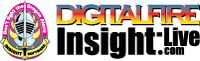•The secret to cool bodies and glazes is a lot of testing.
•The secret to know what to test is material and chemistry knowledge.
•The secret to learning from testing is documentation.
•The place to test, do the chemistry and document is an account at https://insight-live.com
•The place to get the knowledge is https://digitalfire.com

Sign-up at https://insight-live.com today.

# Cape Cross Bentonite

 Oxide Analysis Formula BaO 0.01% 0.000 CaO 0.84% 0.086 Li2O 0.50% 0.096 MgO 3.71% 0.529 K2O 1.07% 0.065 Na2O 2.37% 0.220 SrO 0.04% 0.002 P2O5 0.01% 0.000 TiO2 0.01% 0.001 Al2O3 19.10% 1.077 SiO2 65.30% 6.247 Cr2O3 0.01% 0.000 Fe2O3 0.02% 0.001 LOI 6.26 SO3 0.01 Oxide Weight 534.68 Formula Weight 570.44
If this formula is not unified correctly please contact us.

Out Bound Links

• (Materials - Parent) Bentonite

Montmorillonite, Bentonite USA

• (Suppliers - Manufacturer) [histaddcheckbox] Usakos Wollastonite Mines
• (Typecodes) 1: CLO - Clay Other

## XML for Import into INSIGHT

<?xml version="1.0" encoding="UTF-8"?> <material name="Cape Cross Bentonite" descrip="" searchkey="" loi="0.00" casnumber="52623-66-2"> <oxides> <oxide symbol="BaO" name="Barium Oxide, Baria" status="" percent="0.010" tolerance=""/> <oxide symbol="CaO" name="Calcium Oxide, Calcia" status="" percent="0.840" tolerance=""/> <oxide symbol="Li2O" name="Lithium Oxide, Lithia" status="" percent="0.500" tolerance=""/> <oxide symbol="MgO" name="Magnesium Oxide, Magnesia" status="" percent="3.710" tolerance=""/> <oxide symbol="K2O" name="Potassium Oxide" status="" percent="1.070" tolerance=""/> <oxide symbol="Na2O" name="Sodium Oxide, Soda" status="" percent="2.370" tolerance=""/> <oxide symbol="SrO" name="Strontium Oxide, Strontia" status="" percent="0.040" tolerance=""/> <oxide symbol="P2O5" name="Phosphorus Pentoxide" status="" percent="0.010" tolerance=""/> <oxide symbol="TiO2" name="Titanium Dioxide, Titania" status="" percent="0.010" tolerance=""/> <oxide symbol="Al2O3" name="Aluminum Oxide, Alumina" status="" percent="19.100" tolerance=""/> <oxide symbol="SiO2" name="Silicon Dioxide, Silica" status="" percent="65.300" tolerance=""/> <oxide symbol="Cr2O3" name="Chrome Oxide" status="" percent="0.010" tolerance=""/> <oxide symbol="Fe2O3" name="Iron Oxide, Ferric Oxide" status="" percent="0.020" tolerance=""/> </oxides> <volatiles> <volatile symbol="LOI" name="Loss on Ignition" percent="6.260" tolerance=""/> <volatile symbol="SO3" name="Sulfur Trioxide" percent="0.010" tolerance=""/> </volatiles> </material>

## Feedback, Suggestions

Your email address

Subject

Your Name

Message

Copyright 2003, 2008, 2015 https://digitalfire.com, All Rights Reserved